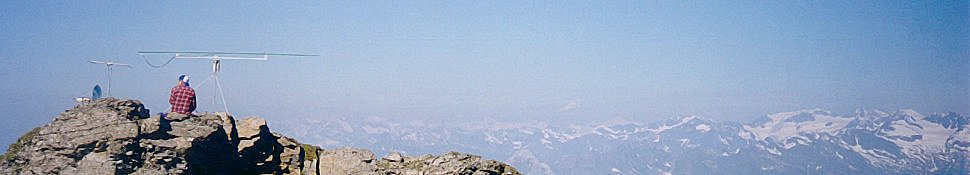# One-turn loop inductance calculation

The high-frequency inductance of single-turn loops of various shapes made of round wire can be estimated accurately with the simplified formulas found in ; accuracy improves when the perimeter is large compared to the wire diameter and is claimed to be within 0.5 percent "for ordinary loop shapes".
There is also another form available to compute the inductance of a rectangular loop.

## INPUT DATA

```Loop perimeter, l :  m

Wire diameter,  d :  mm

Loop shape        :
Circle
Regular octagon
Regular hexagon
Regular pentagon
Square
Equilateral triangle
Isosceles right-angled triangle

```

## CALCULATED VALUES

```Inductance Value, L :  µH
```

References:

  F.E. Terman, "Radio Engineers' Handbook," London, McGraw-Hill, 1st ed., Sep. 1950.